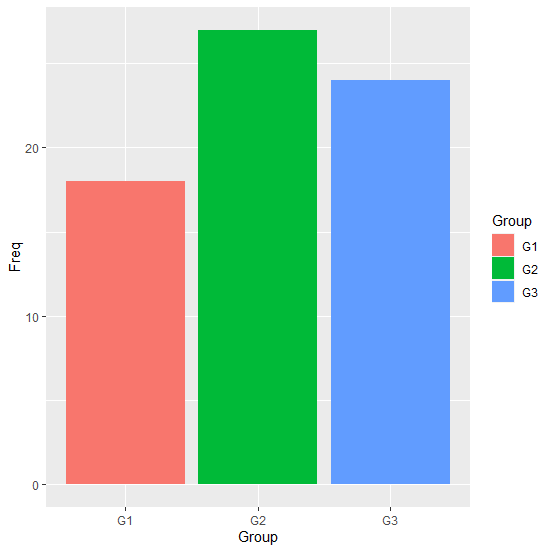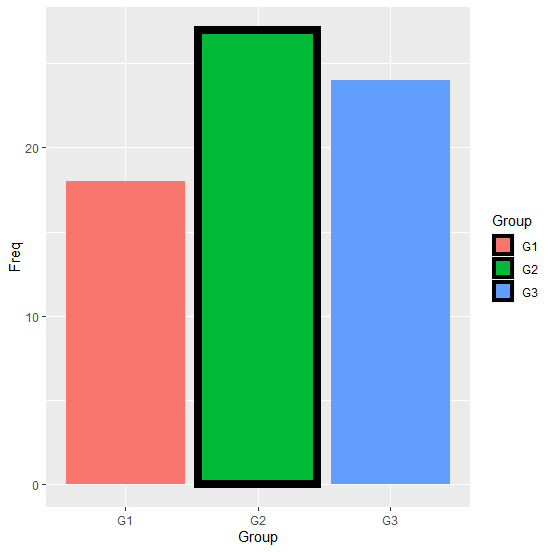# How to create a bar plot using ggplot2 with one bar having black border in R?

The bar plot can be easily created with the help of geom_bar. But if we want to have a different border for a particular bar then we first need to create the bar plot and store it in an object. After that we need to add the original plot with the bar for which we want to have a black border. Check out the below example to understand how it can be done.

## Example

Consider the below data frame:

Live Demo

> Group<-c("G1","G2","G3")
> Freq<-c(18,27,24)
> df<-data.frame(Group,Freq)
> df

## Output

 Group Freq
1 G1   18
2 G2   27
3 G3   24

## Example

> library(ggplot2)
> p<-ggplot(df,aes(Group,Freq,fill=Group))+geom_bar(stat="identity")
> p

## Output:Adding the bar plot with bar having black color border:

## Example

> p+geom_bar(data=df[(df\$Group=="G2"),],stat="identity",
+ aes(Group),size=3,color="black")

## Output: